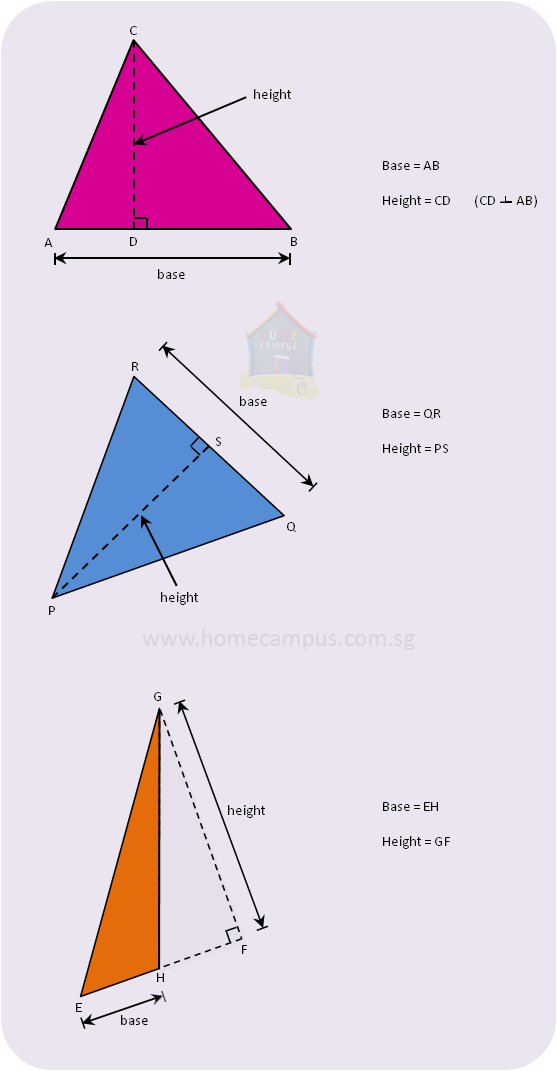## Measurement: Triangle Base and Height

Practice Unlimited Questions

#### 1. Identifying the base and height of a triangle.

What is a base?
Base means bottom.
What is height?
The height of any object is how much it measures from its top to its bottom.
How do you identify the base of a triangle?
Any of the three sides of a triangle can be considered the base of the triangle.
How do you identify the height of a triangle?
The height of a triangle is the perpendicular line dropped onto its base from the corner opposite the base.
Notes:
1.   The height of a triangle corresponds to its base. If the base changes, so does the height.
2.   The height of a triangle is the shortest line onto the base from its opposite corner.
3.   The height of a triangle may be outside the triangle.

#### 2. Following are some triangles with their base and corresponding height indicated on them.